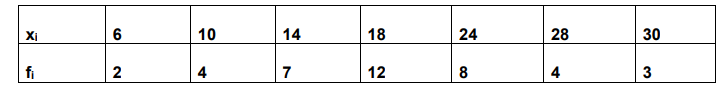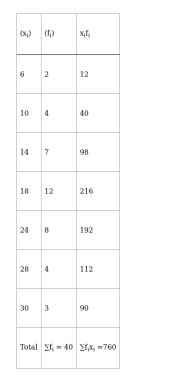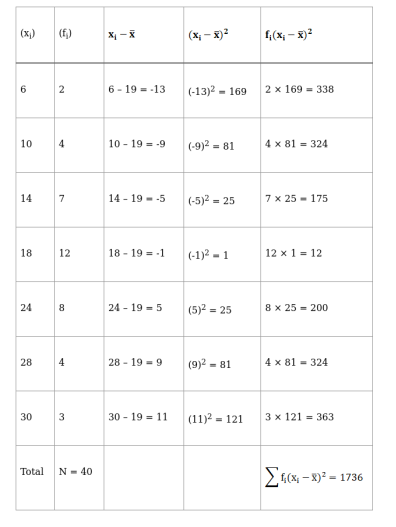Deepak Scored 45->99%ile with Bounce Back Crack Course. You can do it too!

# Using short cut method, find the mean, variation and standard deviation for the data :

Question:

Using short cut method, find the mean, variation and standard deviation for the data :Solution:

To find: MEANNow, $\operatorname{Mean}(\overline{\mathrm{x}})=\frac{\sum \mathrm{f}_{\mathrm{i}} \mathrm{x}_{\mathrm{i}}}{\sum \mathrm{f}_{\mathrm{i}}}$

$=\frac{760}{40}$

$=19$

To find: VARIANCEVariance, $\sigma^{2}=\frac{\sum \mathrm{f}_{\mathrm{i}}\left(\mathrm{x}_{\mathrm{i}}-\overline{\mathrm{x}}\right)^{2}}{\mathrm{~N}}$

$=\frac{1736}{40}$

$=43.4$

To find: STANDARD DEVIATION

Standard Deviation $(\sigma)=\sqrt{\text { Variance }}$

$=\sqrt{43.4}$

$=6.58$